## 4.11Transform condition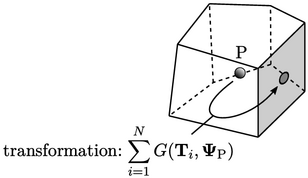Some boundary conditions represent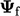at the boundary as a transformation of the cell value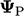. They can be expressed in terms of a general transform condition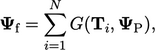(4.13)
where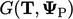is the geometric transformation of variableby a tensor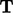. The transformation is calculated as follows: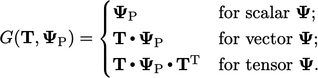(4.14)
When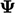is a scalar, the transform condition is equivalent to a zero gradient condition. Otherwise, it is implemented so that terms inin Eq. (4.13 ) contribute to coeﬃcients in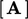. These contributions are implicit which improves convergence when solving the matrix equation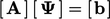.

A factor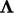is introduced to specify the contribution to internal coeﬃcients. It represents a single internal coeﬃcient for each component of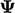so has the same rank as, i.e. it is a vector whenis a vector, and a tensor whenis a tensor.

The multiplication of each coeﬃcient ofby its respective component of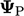is denoted by by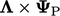. In the case of vector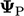, this “component multiplication” is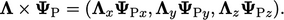For advection discretisation, the face value is represented as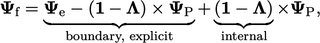(4.15)
where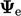is an explicit boundary value, calculated from the expression in Eq. (4.13 ) using the current values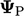. The other explicit term uses the currentwith “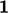” denoting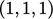for a vector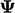and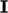for a tensor.

Laplacian discretisation requires the face normal gradient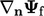. Combining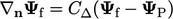with Eq. (4.15 ) gives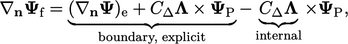(4.16)
where the explicit gradient is calculated by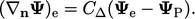(4.17)
The transform condition is summarised within the table below by the value and gradient contributions.

 factor transform value internal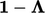value boundary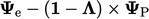gradient internal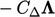gradient boundary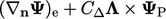Notes on CFD: General Principles - 4.11 Transform condition